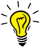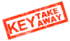GMAT Question of the Day - Daily to your Mailbox; hard ones only

 It is currently 19 Oct 2019, 05:34GMAT Club Daily Prep

Thank you for using the timer - this advanced tool can estimate your performance and suggest more practice questions. We have subscribed you to Daily Prep Questions via email.

Customized
for You

we will pick new questions that match your level based on your Timer History

Track

every week, we’ll send you an estimated GMAT score based on your performance

Practice
Pays

we will pick new questions that match your level based on your Timer History

Not interested in getting valuable practice questions and articles delivered to your email? No problem, unsubscribe here.If n is the product of the integers from 1 to 8, inclusive

 new topic post reply Question banks Downloads My Bookmarks Reviews Important topics
Author Message
TAGS:

Hide Tags

Math ExpertV
Joined: 02 Sep 2009
Posts: 58449
If n is the product of the integers from 1 to 8, inclusive  [#permalink]

Show Tags

2
1600:00

Difficulty:5% (low)

Question Stats:91% (00:50) correct9% (01:05) wrongbased on 2275 sessions

HideShow timer Statistics

If n is the product of the integers from 1 to 8, inclusive, how many different prime factors greater than 1 does n have?

(A) four
(B) five
(C) six
(D) seven
(E) eight

Diagnostic Test
Question: 18
Page: 22
Difficulty: 500

_________________
e-GMAT RepresentativeV
Joined: 04 Jan 2015
Posts: 3074
Re: If n is the product of the integers from 1 to 8, inclusive  [#permalink]

Show Tags

9
8
In this question, I have noticed that many students are prime factorizing every term in the product to find out the answer. But is that necessary?

What if the expression was Z = 1*2*3*…*30. Would you have factorized every term?Let's do a quick concept recap.Concept Recap: Primes are the basic building blocks for every positive integer greater than 1. Every positive integer greater than 1 is itself a prime or a product of primes less than the number itself.

How is this related to the question?: Take the example of 6!. 6! as we all know is equal to $$1*2*3*4*5*6$$. Obviously, we don't need to factorize every element in this expression to find out the different prime factors of 6!. Using the knowledge from the above concept recap that the different prime factors of 6! will be simply the prime numbers less than or equal to 6 itself, we can say the prime factors of 6! are 2, 3 and 5. Therefore 6! has 3 prime factors.

Answer for this question: Primes less than 30 are 2, 3, 5, 7, 11, 13, 17, 19, 23, and 29 (a total of 10 primes.). Therefore, if Z = 30!, then there would 10 different prime factors for Z.In such questions, where you need to find the number of prime factors of a factorial expression, do not waste your time factorizing every term. Number of prime factors of n! will be simply the number of prime numbers less than n.

Footnote for the curious minded: It would have made sense to factorize every term in the expression, if the question had asked the "total number of factors" instead of "number of prime factors". To find the total number of factors, we definitely would need to find the prime factors and their powers in the expression.You can take a stab at the following questions to test your understanding of these concepts.x-is-the-largest-prime-number-less-than-positive-integer-n-p-is-an-in-197329.html

p-is-the-smallest-perfect-cube-greater-than-197336.html

Regards,Krishna
_________________
General Discussion
Math ExpertV
Joined: 02 Sep 2009
Posts: 58449
Re: If n is the product of the integers from 1 to 8, inclusive  [#permalink]

Show Tags

1
3
SOLUTION

If n is the product of the integers from 1 to 8, inclusive, how many different prime factors greater than 1 does n have?

(A) four
(B) five
(C) six
(D) seven
(E) eight

$$n=8!$$, so it has 4 prime factors: 2, 3, 5, and 7.

_________________
ManagerJoined: 07 Sep 2011
Posts: 59
Location: United States
Concentration: Strategy, International Business
GMAT 1: 640 Q39 V38WE: General Management (Real Estate)
Re: If n is the product of the integers from 1 to 8, inclusive  [#permalink]

Show Tags

Difficulty Sub 550

8! has factors of 2, 3, 5 and 7 as 4 and 8 has 2 and six has 3. thus answer is A.
GMAT TutorG
Status: Tutor - BrushMyQuant
Joined: 05 Apr 2011
Posts: 622
Location: India
Concentration: Finance, Marketing
Schools: XLRI (A)
GMAT 1: 700 Q51 V31GPA: 3
WE: Information Technology (Computer Software)
Re: If n is the product of the integers from 1 to 8, inclusive  [#permalink]

Show Tags

2
n = 1*2*3*4*5*6*7*8

so only Prime numbers which will be factors of n will be 2,3,5,7 (as prime numbers which are greater than 7 will not be there in the product of 1 to 8!)

So Answer is A (four)

Hope it Helps!
_________________
ManagerJoined: 26 Dec 2011
Posts: 89
Re: If n is the product of the integers from 1 to 8, inclusive  [#permalink]

Show Tags

Is it correct to say prime factors greater than 1? 1 is not a prime factor at all. If one says prime factor greater than 2, then it does make sense. Am I right in making this statement?
Senior ManagerJoined: 23 Oct 2010
Posts: 323
Location: Azerbaijan
Concentration: Finance
Schools: HEC '15 (A)
GMAT 1: 690 Q47 V38Re: If n is the product of the integers from 1 to 8, inclusive  [#permalink]

Show Tags

yep, the phrase " different prime factors greater than 1" sounds strange, since in fact, all of these primes are different.furthermore, no need to point out about 1, since 1 is not prime.
_________________
Happy are those who dream dreams and are ready to pay the price to make them come true

I am still on all gmat forums. msg me if you want to ask me smth
Senior ManagerStatus: 1,750 Q's attempted and counting
Affiliations: University of Florida
Joined: 09 Jul 2013
Posts: 475
Location: United States (FL)
Schools: UFL (A)
GMAT 1: 600 Q45 V29GMAT 2: 590 Q35 V35GMAT 3: 570 Q42 V28GMAT 4: 610 Q44 V30GPA: 3.45
WE: Accounting (Accounting)
Re: If n is the product of the integers from 1 to 8, inclusive  [#permalink]

Show Tags

1
2
n = 8!

Prime factorization of 8!
= 1, 2, 3, 2*2, 5, 2*3, 7, 2*2*2

= 2, 3, 5, 7

= 4 prime factors > 1
ManagerJoined: 12 Jan 2013
Posts: 142
Re: If n is the product of the integers from 1 to 8, inclusive  [#permalink]

Show Tags

8! = 1x2x3x4x5x6x7x8.. Just do prime factorization of each integer alone and then count the number of different primes in total.
Current StudentD
Joined: 12 Aug 2015
Posts: 2567
Schools: Boston U '20 (M)
GRE 1: Q169 V154Re: If n is the product of the integers from 1 to 8, inclusive  [#permalink]

Show Tags

Here is my solution =>
n=$$8! =>8*7*6*5*4*3*2*1=> 2^7*3^2*5*7$$=> Clearly it has 4 prime factors.
Hence A
(Additionally it has -> 8*3*2*2 =>96 factors )
_________________
Board of DirectorsD
Status: QA & VA Forum Moderator
Joined: 11 Jun 2011
Posts: 4772
Location: India
GPA: 3.5
WE: Business Development (Commercial Banking)
Re: If n is the product of the integers from 1 to 8, inclusive  [#permalink]

Show Tags

Bunuel wrote:
If n is the product of the integers from 1 to 8, inclusive, how many different prime factors greater than 1 does n have?

(A) four
(B) five
(C) six
(D) seven
(E) eight

8! = 8 * 7 * 6 * 5 * 4 * 3 * 2 * 1

There can be only 4 different prime factors greater than 1 , as highlighted in Blue above..

_________________
Thanks and Regards

Abhishek....

PLEASE FOLLOW THE RULES FOR POSTING IN QA AND VA FORUM AND USE SEARCH FUNCTION BEFORE POSTING NEW QUESTIONS

How to use Search Function in GMAT Club | Rules for Posting in QA forum | Writing Mathematical Formulas |Rules for Posting in VA forum | Request Expert's Reply ( VA Forum Only )
InternB
Joined: 30 Mar 2016
Posts: 37
If n is the product of the integers from 1 to 8, inclusive  [#permalink]

Show Tags

Hi e-GMAT

This Takeway: Number of prime factors of n! will be simply the number of prime numbers less than n: is true if n = even

For example:

n = 4 => n! = 1 x 2 x 3 x 4 So, different prime factors of 4! are: 2 & 3 which are less than n = 4

BUT once n = odd, Number of prime factors of n! will be simply the number of prime numbers less than OR EQUAL to n.

For example:

n = 7 => n! = 7! = 1 x 2 x 3 x 4 x 5 x 6 x 7 So different prime factors of 7! = 2, 3, 5, & 7. In this scenario, the prime number 7 is equal to n = 7.

So, the Takeaway would be: Number of prime factors of n! will be simply the number of prime numbers less than OR EQUAL to n.

Is this revised Takeaway correct?

Many thanksTarget Test Prep RepresentativeG
Status: Head GMAT Instructor
Affiliations: Target Test Prep
Joined: 04 Mar 2011
Posts: 2815
Re: If n is the product of the integers from 1 to 8, inclusive  [#permalink]

Show Tags

Bunuel wrote:
If n is the product of the integers from 1 to 8, inclusive, how many different prime factors greater than 1 does n have?

(A) four
(B) five
(C) six
(D) seven
(E) eight

n = 8 x 7 x 6 x 5 x 4 x 3 x 2

We can prime factorize and we have:

n = 2^7 x 3^2 x 5^1 x 7^1

Thus, n has 4 different prime factors.

Alternate solution:

In general, the number of distinct prime factors that k! (where k > 1) has is the number of prime numbers less than or equal to k. We have n = 8!, so k = 8; the number of prime numbers less than or equal to 8 is 4, namely, 2, 3, 5 and 7.

_________________

Jeffrey Miller

Head of GMAT Instruction

Jeff@TargetTestPrep.com

See why Target Test Prep is the top rated GMAT quant course on GMAT Club. Read Our Reviews

If you find one of my posts helpful, please take a moment to click on the "Kudos" button.

InternB
Status: Don't watch the clock,Do what it does, Keep Going.
Joined: 10 Jan 2017
Posts: 37
Re: If n is the product of the integers from 1 to 8, inclusive  [#permalink]

Show Tags

Prime factors are those numbers which are only divisible by one and itself.prime factors between 1 to 8 inclusive are 2,3,5,7. So, answer is 4.
Non-Human UserJoined: 09 Sep 2013
Posts: 13271
Re: If n is the product of the integers from 1 to 8, inclusive,  [#permalink]

Show Tags

Hello from the GMAT Club BumpBot!

Thanks to another GMAT Club member, I have just discovered this valuable topic, yet it had no discussion for over a year. I am now bumping it up - doing my job. I think you may find it valuable (esp those replies with Kudos).

Want to see all other topics I dig out? Follow me (click follow button on profile). You will receive a summary of all topics I bump in your profile area as well as via email.
_________________Re: If n is the product of the integers from 1 to 8, inclusive,   [#permalink] 18 Sep 2019, 06:04
Display posts from previous: Sort by

If n is the product of the integers from 1 to 8, inclusive

 new topic post reply Question banks Downloads My Bookmarks Reviews Important topics

 Powered by phpBB © phpBB Group | Emoji artwork provided by EmojiOne